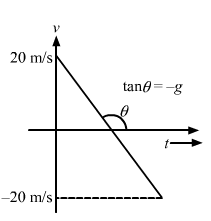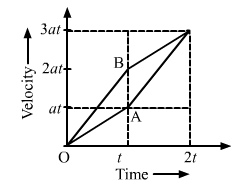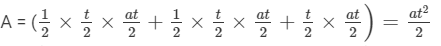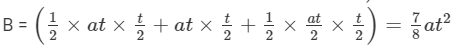NEET  >  HC Verma Solutions: Kinematics- Rest and Motion

# HC Verma Solutions: Kinematics- Rest and Motion | Physics Class 11 - NEET

 1 Crore+ students have signed up on EduRev. Have you?

Page No. 48
Ques 1: Galileo was punished by the Church for teaching that the sun is stationary and the earth moves around it. His opponents held the view that the earth is stationary and the sun moves around it. If the absolute motion has no meaning, are the two viewpoints not equally correct or equally wrong?
Ans:
The absolute motion has no meaning. In the relative motion view, the two viewpoints are the same. Hence, both viewpoints are equally correct or equally wrong.

Ques 2: When a particle moves with constant velocity, its average velocity, its instantaneous velocity and its speed are all equal. Comment on this statement.
Ans:
Constant velocity means that a particle has the same direction and speed at every point. So, its average velocity and instantaneous velocity are equal. Its speed being a scalar quantity is equal in magnitude only.

Ques 3: A car travels at a speed of 60 km/hr due north and the other at a speed of 60 km/hr due east. Are the velocities equal? If no, which one is greater? If you find any of the questions irrelevant, explain.
Ans:
No, as the directions of the cars are different, their velocities are not equal, as velocity is a vector quantity.
We cannot compare velocities on the basis of direction only, so the question is irrelevant.

Ques 4: A ball is thrown vertically upward with a speed of 20 m/s. Draw a graph showing the velocity of the ball as a function of time as it goes up and then comes back.
Ans:Ques 5: The velocity of a particle is towards west at an instant. Its acceleration is not towards west, not towards east, not towards north and towards south. Give an example of this type of motion.
Ans:
Motion of a projectile at the highest point of its path: Because at this point, the projectile has only horizontal component of velocity. So, it can have velocity towards west and acceleration in the vertically downward direction.

Ques 6: At which point on its path a projectile has the smallest speed?
Ans: A projectile has the lowest speed at the highest point of its path because the vertical component of velocity is zero at this point

Ques 7: Two particles A and B start from rest and move for equal time on a straight line. The particle A has an acceleration a for the first half of the total time and 2a for the second half. The particle B has an acceleration 2a for the first and a for the second half. Which particle has covered larger distance?
Ans:From the velocity–time graph, we have:
Distance covered byDistance covered by∴ Distance covered by A < Distance covered by B

Ques 8: If a particle is accelerating, it is either speeding up or speeding down. Do you agree with this statement?
Ans: Acceleration does not mean speeding up or speeding down. It means the change of velocity either in direction or in magnitude.

Ques 9: A food packet is dropped from a plane going at an altitude of 100 m. What is the path of the packet as seen from the plane? What is the path as seen from the ground? If someone asks "what is the actual path", what will you answer?
Ans: The path of the packet (as seen from the plane) is a vertically downward straight line, as the horizontal velocity of the packet and the plane is the same.
As seen from the ground, the path of the packet is a parabola.
The path is defined with respect to some reference frame. As there is no absolute reference frame, no actual path is defined.

Ques 10: Give examples where (a) the velocity of a particle is zero but its acceleration is not zero, (b) the velocity is opposite in direction to the acceleration, (c) the velocity is perpendicular to the acceleration.
Ans: (a) At the highest point when a particle is thrown vertically upwards.
(b) While going up when a particle is thrown vertically upwards.
(c) At the highest point of a full projectile.

The document HC Verma Solutions: Kinematics- Rest and Motion | Physics Class 11 - NEET is a part of the NEET Course Physics Class 11.
All you need of NEET at this link: NEET

## Physics Class 11

124 videos|464 docs|210 tests

## Physics Class 11

124 videos|464 docs|210 tests

### How to Prepare for NEET

Read our guide to prepare for NEET which is created by Toppers & the best Teachers

Track your progress, build streaks, highlight & save important lessons and more!(Scan QR code)

,

,

,

,

,

,

,

,

,

,

,

,

,

,

,

,

,

,

,

,

,

;